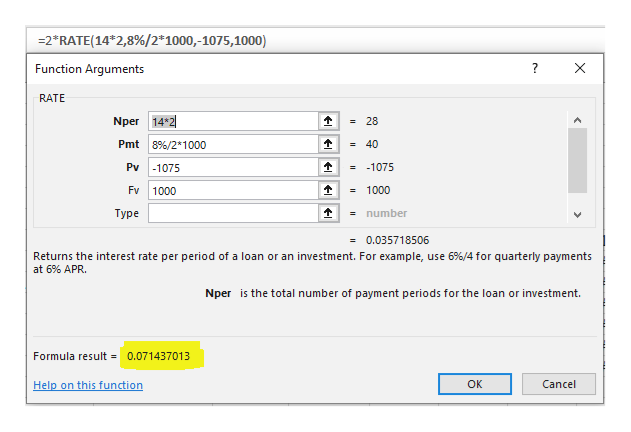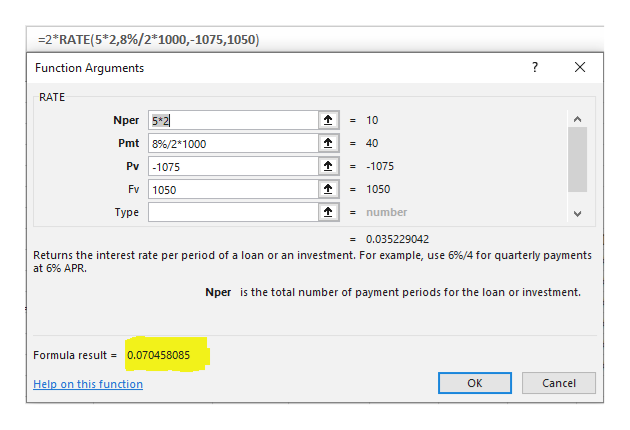# A corporate bond matures in 14 years.  The bond has an 8% semiannual coupon and a par value of \$1,000.  The bond is callable in five years at a call price of \$1,050. The price of the bond today is \$1,075.  What are the bond’s yield to maturity and yield to call?

Question
112 views

A corporate bond matures in 14 years.  The bond has an 8% semiannual coupon and a par value of \$1,000.  The bond is callable in five years at a call price of \$1,050. The price of the bond today is \$1,075.  What are the bond’s yield to maturity and yield to call?

check_circle

Step 1

Calculate the yield to maturity as follows:

MS-Excel --> Formulas --> Financials --> Ratehelp_outlineImage Transcriptionclose2*RATE(14 2,8%/2*1000,-1075, 1000) Function Arguments X RATE Nper 14*2 = 28 Pmt 8%/2* 1000 =40 Pv -1075 = -1075 Fv 1000 =1000 = number Турe =0.035718506 Returns the interest rate per period of a loan or an investment. For example, use 6 % / 4 for quarterly payments at 6% APR. Nper is the total number of payment periods for the loan or investment. Formula result =0.071437013 Cancel Help on this function ок fullscreen
Step 2

Therefore, the yield to maturity is 7.14%.

Step 3

Calculate the yield to call as follows:

MS-Excel --&g...help_outlineImage Transcriptionclose2*RATE(5*2,8%/2*1000,-1075,1050) Function Arguments X RATE Nper 5*2 =10 Pmt 8%/2* 1000 =40 = -1075 Pv 1075 Fv 1050 =1050 Туре =number =0.035229042 Returns the interest rate per period of a loan or an investment. For example, use 6% / 4 for quarterly payments at 6% APR Nper is the total number of payment periods for the loan or investment. Formula result 0.070458085 Help on this function ок Cancel fullscreen

### Want to see the full answer?

See Solution

#### Want to see this answer and more?

Solutions are written by subject experts who are available 24/7. Questions are typically answered within 1 hour.*

See Solution
*Response times may vary by subject and question.
Tagged in